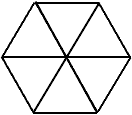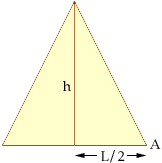Name: Carl Who is asking: Student Level: Secondary Question: What is the formula for finding the area of a regular Hexagon and a regular Pentagon Hi Carl, I'm not going to give you a formula but I will show you how to find the area. First join each vertex of the regular polygon to its center as in the diagram belowTo find the area of the regular polygon first find the area of one of the triangles and then multiply by the number of sides in the polygon. Suppose that the polygon has n sides each of length L. Each of the triangles is isosceles and the angle at the vertex is  360o/n. One such triangle is in the diagram below.Since the triangle is isosceles and you know the angle at the vertex, you can find the angle measurement of the angle at A in the diagram. Thus h is  L/2 x tan(A) and the area of the triangle is  1/2 L h. I hope this helps, Penny Go to Math Central